Determination of price and quantity supplied by monopolistic firm in the short run

This article describes the process by which a monopoly firm, i.e., a firm that is the only firm selling a particular commodity, selects the quantity to produce and the price to set for the commodity.

Here are some key features of a monopoly firm:

1. The firm is a price setter rather than a price taker -- it can choose any price it wants.
2. However, for a given price set by the firm, and a given quantity that the firm produces, there is no guarantee that the firm will be able to sell all of what it produces. Thus, instead of being told a market price and trying to optimize the quantity, the firm needs to optimize a (price,quantity) pair based on the market demand curve.

The situation is in sharp contrast to that of determination of quantity supplied by firm in perfectly competitive market in the short run.

The situation is similar to determination of quantity supplied by firm in oligopoly in the short run. In the latter, however, the firm also has to be concerned about the behavior of competing firms, and the response these firms may show to price changes by the firm. Oligopolistic competition is typically studied using tools of game theory.

More generally, when a firm has market power, it has some leeway in setting prices but, in return, has to explicitly consider the market demand curve it faces. When a firm has monopoly, the leeway in setting prices as well as the importance of the market demand curve are maximal.

The overall conclusion will be the following:

The absolute (i.e., global) maximum for profit must occur either at one of these three points: zero quantity of production, the maximum quantity of production, or some local maximum at an intermediate quantity of production. In the last case, that must be a point where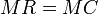$MR = MC$ and, more specifically, the value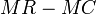$MR - MC$ is changing sign from positive to negative. Here,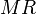$MR$ and$MC$ denote respectively the marginal revenue and marginal cost.

To jump directly to the meat of the analysis, see the formal mathematical analysis section.

Similar determinations

Contrast with firm in perfectly competitive market

There are three key differences. The first two are related and the third follows from them:

Parameter Firm in perfectly competitive market Monopolistic firm
control over market price no control. The firm must accept the market price. significant control. The firm sets both its price and quantity of production.
guarantee of selling everything it produces guaranteed, as long as it prices at the market price. no guarantee. The firm needs to choose its price appropriately based on its estimate of the nature of demand and the quantity it produces.
nature of marginal revenue curve (marginal revenue plotted on the vertical axis as a function of quantity produced on the horizontal axis) flat horizontal curve with price coordinate equal to the market price it's complicated, but generally, a downward sloping curve. It can be determined algebraically from the demand curve of the firm -- it has a marginal-to-average relationship with that curve.

Another way of putting these is that monopolistic firms have more power (in the sense of setting prices) but also more responsibility (in the sense of having to figure what price to set).

Most of the analysis here can be transferred to the perfectly competitive case, with the following change: the marginal revenue curve is replaced by a horizontal curve at value equal to the market price.

Law of one price assumption and price discrimination

In the discussion here, we assume the law of one price, i.e., that the firm must offer a single price to all those buying from it. In other words, we assume that it is impossible for the firm to practice price discrimination, i.e., charge different prices to different buyers.

If the firm can practice price discrimination, the analysis changes considerably. The key significance is that with price discrimination, the firm can charge higher prices for buyers with higher reservation prices and thus avoid to some extent the trade-off between expanding its market and lowering its price.

Cost and revenue definitions

We assume that the firm has two costs:

• Fixed costs are fixed in advance and cannot be altered in the short run. These may include investments in land or other fixed assets that cannot be acquired or got rid of quickly.
• Variable costs are costs that vary with the quantity produced.

We define:

Cost or revenue notion Acronym Definition How does it vary with quantity?
Fixed cost FC Cost of expenditure on fixed factors of production that cannot be varied in the short run Independent of quantity
Variable cost VC Cost of expenditure on factors of production that can be varied in the short run Increases with quantity
Total cost TC Fixed cost + Variable cost Increases with quantity
Average cost ATC, AC (Total cost)/Quantity Generally, first decreases and then increases with quantity
Average fixed cost AFC (Fixed cost)/Quantity Decreases with quantity (by definition, because denominator is increasing and numerator is constant).
Average variable cost AVC (Variable cost)/Quantity Generally, increases with quantity when quantity is large enough. For small quantities, it may decrease with quantity.
Marginal cost MC Derivative of total cost with respect to quantity; since fixed cost is independent of quantity, this is the same as the derivative of variable cost with respect to quantity Generally, increases with quantity when quantity is large enough. For small quantities, it may decrease with quantity.
Revenue R Revenue earned by producing a given quantity of the good. It equals the product of quantity produced and the (unit) market price. Note that because of the small size of the firm relative to the market, it must sell everything it produces at the market price Increases with quantity (by definition).
Price P Price chosen by the firm See discussion below
Marginal revenue MR Derivative of total revenue with respect to quantity. Depends on quantity
Profit (not accounting for fixed costs) Equals R - VC, same as Q(P - AVC) where Q is the quantity produced, P is the unit price chosen by the firm, and AVC is the average variable cost at the given value of Q.
Profit (accounting for fixed costs) Equals R - TC, same as Q(P - ATC), where Q is the quantity produced, P is the unit price, and AC is the average (total of fixed and variable) cost. In the short run, the firm may choose to produce even if the profit, after accounting for fixed costs, is negative.

Calculation problem for the firm

Information available to the firm

As before, we assume that the firm serves an entire market and has no competing firms. The firm has two pieces of information:

Piece of information Nature Equivalent pieces of information How it's decided How the firm gets to know it
the marginal cost curve of the firm This is a curve describing the marginal cost as a function of quantity produced. Traditionally, the quantity is plotted along the horizontal axis and the cost function along the vertical axis. It is not a single number but rather a functional relationship. Knowledge of the marginal cost curve is mathematically equivalent to knowledge of the average variable cost curve, the variable cost curve, and (subject to knowledge of the fixed cost),the average fixed cost curve, the average total cost curve, and the total cost curve. This means that knowledge of any one of these curves can be used to deduce the others. Determined by the firm's production technology and factors of production. The curve itself is not subject to change in the short run. The firm may know this curve through explicit theoretical knowledge of its production process, or it may collect the data through experimentation with different production quantities.
the market demand curve facing the firm This is the market demand curve that plots the quantity demanded by the market the firm serves as a function of the price. See determinants of demand for details. See demand curve estimation for details.

Goal of the firm

The firm has to choose two things:

• The quantity that it produces. Call this$Q$.
• The price at which it sells whatever it produces. Call this$P$.

The goal of the firm is to maximize its profit. Assuming that the firm is able to sell everything it makes, the profit (not accounting for fixed costs) is given by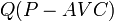$Q(P - AVC)$ where$Q$ is the quantity produced,$P$ is the price chosen by the firm, and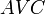$AVC$ is the average variable cost (dependent on$Q$).

Note on conventions for profit: accounting and not accounting for fixed costs

If$Q$ denotes the quantity produced,$P$ denotes the market price (which is also the same as the marginal and average revenue) then the total revenue is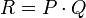$R = P \cdot Q$, and the total variable cost is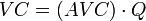$VC = (AVC) \cdot Q$ where$AVC$ is the average variable cost for the given choice of$Q$. The net profit (not accounting for fixed costs) is$R - VC$, which becomes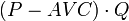$(P - AVC) \cdot Q$.

In particular, the net profit (not accounting for fixed costs) is zero at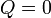$Q = 0$.

The net profit accounting for fixed costs is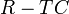$R - TC$, which becomes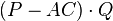$(P - AC) \cdot Q$, where$AC$ is the average total cost, including both average fixed costs and average variable costs.

This is smaller than the profit calculated by not accounting for fixed costs. Also, for the short-run choices, this profit value may well be negative, because the firm treats its fixed costs as sunk costs.

Note that as far as the maximization problem is concerned, both choices of the profit function are equivalent, because they differ by a value that is constant (in the short run). However, there is an advantage to choosing the convention where we compute profits not accounting for fixed costs. The advantage is that it computes the profits relative to the situation of staying idle, i.e., producing nothing, and hence, can be more useful in quickly figuring out whether a certain quantity of production is more profitable than producing nothing.

Choice of price given quantity

The first step in the firm's analysis is to reduce the number of choices from two variables (quantity and price) to one (quantity only). This is easy to do. For a given choice of quantity$Q$, the firm wants to choose a price$P$ so that$Q(P - AVC)$ is as large as possible, subject to the condition that the firm sells everything it produces. Assuming the law of demand (i.e., a downward sloping demand curve) it is easy to see that the largest possible such price is a price such that the (price,quantity) point lies on the market demand curve. In other words, once the firm has chosen the quantity, the price is automatically determined as being the corresponding price point for the market demand curve. Conversely, if the firm chooses a price, the corresponding quantity is the quantity demanded at that price as per the market demand curve.

Thus, we have reduced the firm's choices from two variables to one (by linking the two variables via the market demand curve). What's left is to choose the quantity produced so as to maximize the firm's profits.

Definition of marginal revenue

Now that we have determined the firm's price as a function of the quantity produced, the firm's total revenue ($PQ$) also becomes a function of the quantity produced. Thus, we can take the derivative of total revenue with respect to quantity produced. This derivative is called marginal revenue and is abbreviated MR. Geometrically, the marginal revenue is the derivative with respect to the quantity produced of the area of the rectangle between the axes and the (quantity, price) point on the demand curve.

Note that marginal revenue depends, not on the firm's production technologies, but rather, completely on the firm's market demand curve. Moreover, in analogy terms:

Marginal revenue curve : Market demand curve :: Marginal cost curve : Average variable cost curve

Relationship between marginal revenue curve and market demand curve

The marginal revenue curve lies below the market demand curve everywhere. This follows from the law of demand: the demand curve is downward-sloping. In particular, this means that increasing the quantity demanded requires reducing the price somewhat. The derivative of total revenue with respect to quantity is therefore less than the price.

The extent to which the marginal revenue curve diverges from the market demand curve is directly related to the extent of deadweight loss created in the situation.

Relationship between marginal revenue and price-elasticity of demand

Further information: price-elasticity of demand

The relationship is below:

Condition on price-elasticity of demand Conclusion for marginal revenue (note that this is the derivative with respect to quantity, not price. The sign conclusion is opposite if we are trying to figure out the rate of change of revenue with respect to price) Verbal explanation
zero undefined/infinite vertical demand curve, meaning that a slight increase in quantity leads to an infinitely quick decrease in price.
between 0 and -1 (in magnitude, between 0 and 1) negative the quantity demanded is highly price-insensitive. Thus, the price is highly sensitive to the quantity demanded (by the inverse function theorem, the sensitivies are reciprocal). A small increase in quantity demanded leads to a larger compensating decrease in the price that can be charged to sell out the quantity, causing the total revenue to decrease.
equal to -1 zero (total revenue remains constant). Here price and quantity are inversely proportional to each other.
less than -1 (in magnitude, greater than 1) positive the quantity demanded is highly price-sensitive. This, the price is insensitive to the quantity demanded. A small increase in quantity demanded leads to a smaller compensating decrease in the price that can be charged to sell out the quantity, causing the total revenue to increase.
infinite positive, equal to the price horizontal demand curve, which means that there is a fixed price and the marginal revenue equals that price.

There is no short run supply curve

For the corresponding determination of quantity supplied by firm in perfectly competitive market in the short run, it is possible to construct a short run supply curve by finding the profit-maximizing quantity produced by the firm for each price, and expressing this functional relationship through the curve. Here, the price is coming from the market.

A monopolistic firm does not receive a price from the market. Rather, the firm receives a market demand curve and chooses both a price and quantity of production. Thus, there is no notion of a short run supply curve for a monopolistic firm, because it is not receiving a price.

It is still relevant, for a monopolistic firm, to consider the question of how shifts (expansions or contractions) in the demand curve affect the price and quantity produced. However, the analysis must revert directly to the calculation problem for the monopolistic firm and cannot use the short run supply curve as a visual aid.

Formal mathematical analysis

Determining the profit-maximizing quantity of production

We make the following assumptions:

• The firm can choose to produce any quantity between$0$ and a maximum quantity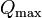$Q_{\max}$.
• The marginal cost$MC$ is defined at all quantities of production in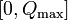$[0,Q_{\max}]$. Formally, it is the derivative of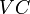$VC$ with respect to$Q$, though at the endpoints$0$ and$Q_{\max}$, we take one-sided derivatives.
• For simplicity, we also assume that the$MC$ curve is continuous and is not highly oscillatory, i.e., we can break up the interval$[0,Q_\max]$ into finitely many pieces and the curve is either increasing or decreasing on each piece.

Our goal is to prove the following:

The absolute (i.e., global) maximum for profit must occur either at one of these three points: zero quantity of production, the maximum quantity of production, or some local maximum at an intermediate quantity of production. In the last case, that must be a point where$MR = MC$ and, more specifically, the value$MR - MC$ is changing sign from positive to negative.

We establish this formally in a few steps.

Step no. Assertion Explanation
1 The profit function (not accounting for fixed costs) is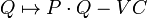$Q \mapsto P \cdot Q - VC$ where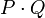$P \cdot Q$ is the product of the market price$P$ and the quantity produced$Q$. Profit (not accounting for fixed costs) is total revenue minus variable cost. Total revenue is the product$PQ$.
2 The derivative of the profit function with respect to$Q$ is$MR - MC$ where$MR$ is the marginal revenue (defined as the derivative of total revenue with respect to quantity, using price as a function of quantity via the market demand curve) and$MC$ is the marginal cost (dependent on$Q$). [SHOW MORE]
3 For those quantities of production where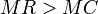$MR > MC$, the profit function is increasing with respect to$Q$. For those quantities of production where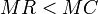$MR < MC$, the profit function is decreasing with respect to$Q$. See positive derivative implies increasing, negative derivative implies decreasing.
4 The local maxima for the profit function must occur at points where$MR = MC$. Further, under reasonable assumptions of the function not being heavily oscillatory, we must have$MR > MC$ for$Q$ slightly less than the point of local maximum and$MR < MC$ for$Q$ slightly more than the point of local maximum. In other words, the$MC$ curve is overtaking the$MR$ curve. Follows from (3). We can also interpret this in terms of the first derivative test applied to the profit function as a function of$Q$.
5 The absolute (i.e., global) maximum for profit must occur either at one of these three points: zero quantity of production, the maximum quantity of production, or some local maximum at an intermediate quantity of production. In the last case, that must be a point where$MR = MC$ and the$MC$ curve is overtaking the$MR$ curve. Follows directly from Step (4).

Graphical interpretation of revenue and profit

We have the following:

• The revenue is given by the product of the price and quantity produced. It is the area of a rectangle with two sides along the axes and the optimal (quantity,price) vertex as the one vertex not on the axes.
• The profit is given by the signed area between the marginal cost curve and the horizontal line for the price, up till the optimal quantity produced. Explicitly, it is: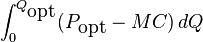$\int_0^{Q_{\mbox{opt}}} (P_{\mbox{opt}} - MC) \, dQ$

where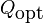$Q_{\mbox{opt}}$ is the optimal quantity and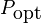$P_{\mbox{opt}}$ is the corresponding price.

Note that, unlike the case of perfect competition, we do not have$P = MC$ at the optimum quantity of production. In other words, at the firm's profit-maximizing choice of price and quantity, its marginal cost is not equal to its price. Rather, the marginal cost is equal to the marginal revenue. This is somewhat less than the price because the marginal revenue curve is below the market demand curve, or more concretely, because increasing the quantity sold requires reducing the price, and this has a compensating downward effect on revenue.